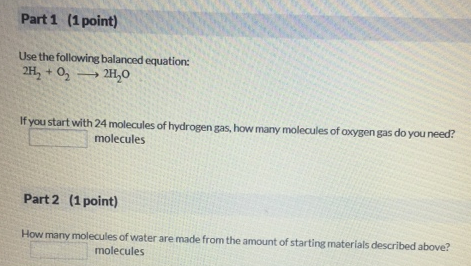# Problem: Part 1Use the following balanced equation: 2H2 + O2 → 2H2O If you start with 24 molecules of hydrogen gas, how marry molecules of oxygen gas do you need?Part 2How many molecules of water are made from the amount of starting materials described above?

###### FREE Expert Solution
92% (340 ratings)###### Problem Details

Part 1

Use the following balanced equation:

2H2 + O2 → 2H2

If you start with 24 molecules of hydrogen gas, how marry molecules of oxygen gas do you need?

Part 2

How many molecules of water are made from the amount of starting materials described above?